Anzeige

# singly-reinforced-beam.ppt

3. Aug 2022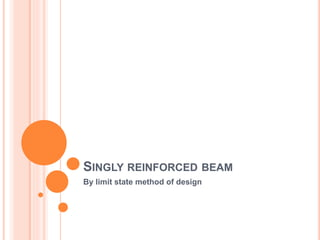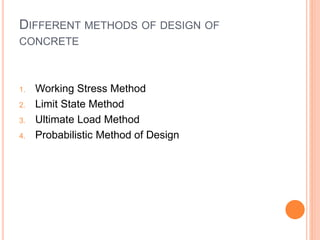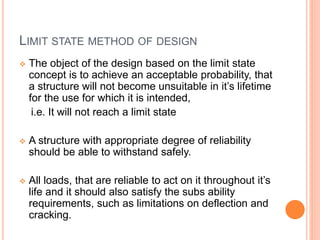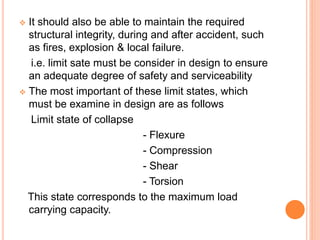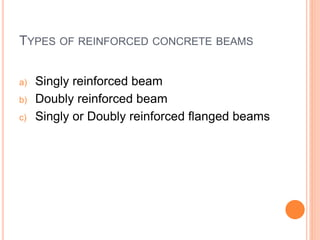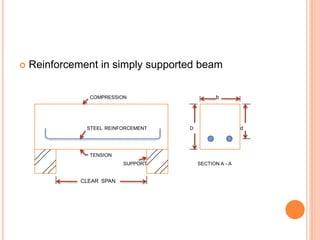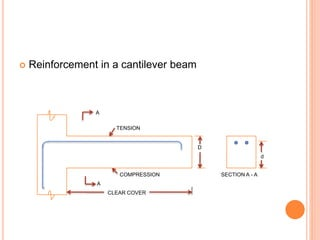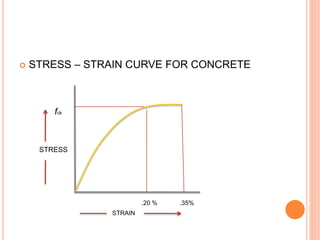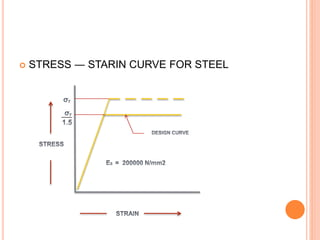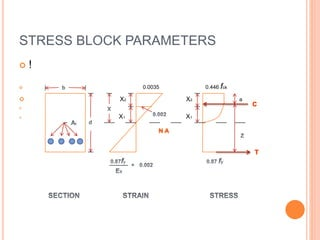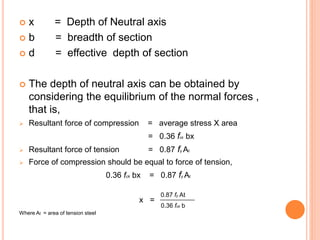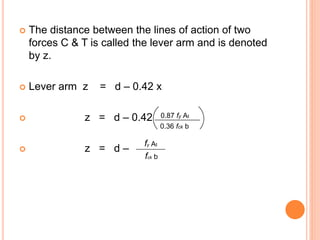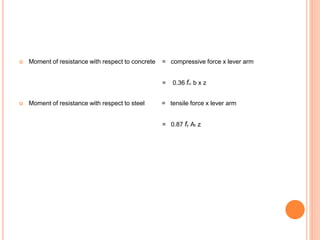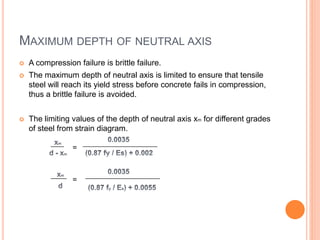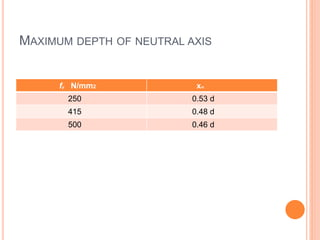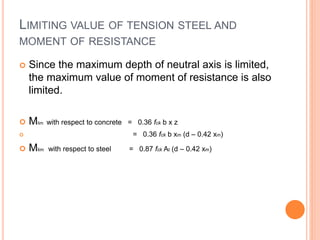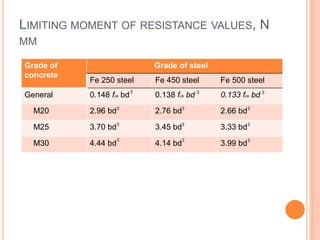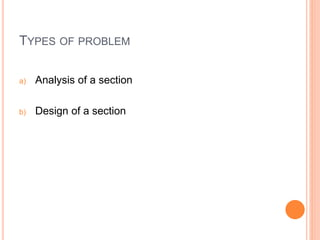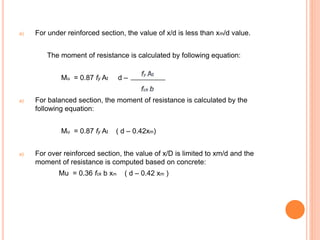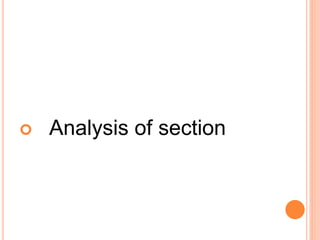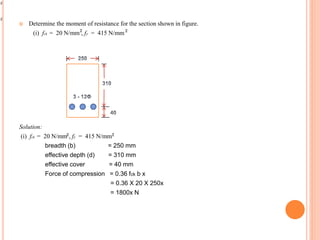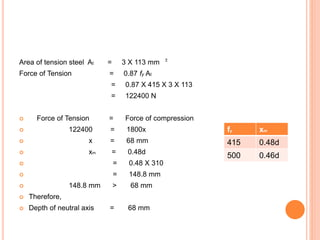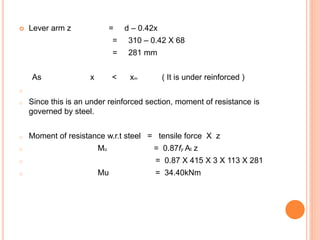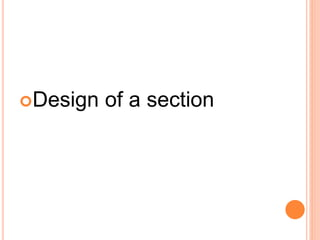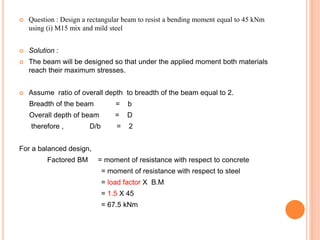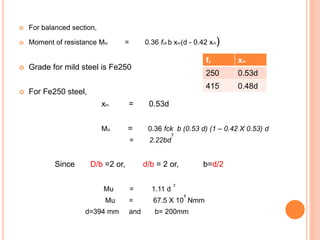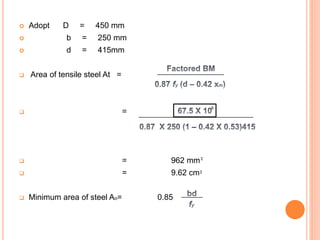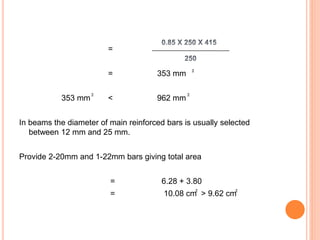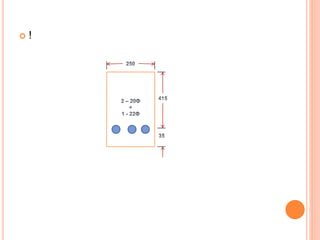1 von 30
Anzeige

### singly-reinforced-beam.ppt

1. SINGLY REINFORCED BEAM By limit state method of design
2. DIFFERENT METHODS OF DESIGN OF CONCRETE 1. Working Stress Method 2. Limit State Method 3. Ultimate Load Method 4. Probabilistic Method of Design
3. LIMIT STATE METHOD OF DESIGN  The object of the design based on the limit state concept is to achieve an acceptable probability, that a structure will not become unsuitable in it’s lifetime for the use for which it is intended, i.e. It will not reach a limit state  A structure with appropriate degree of reliability should be able to withstand safely.  All loads, that are reliable to act on it throughout it’s life and it should also satisfy the subs ability requirements, such as limitations on deflection and cracking.
4.  It should also be able to maintain the required structural integrity, during and after accident, such as fires, explosion & local failure. i.e. limit sate must be consider in design to ensure an adequate degree of safety and serviceability  The most important of these limit states, which must be examine in design are as follows Limit state of collapse - Flexure - Compression - Shear - Torsion This state corresponds to the maximum load carrying capacity.
5. TYPES OF REINFORCED CONCRETE BEAMS a) Singly reinforced beam b) Doubly reinforced beam c) Singly or Doubly reinforced flanged beams
6. SINGLY REINFORCED BEAM  In singly reinforced simply supported beams or slabs reinforcing steel bars are placed near the bottom of the beam or slabs where they are most effective in resisting the tensile stresses.
7.  Reinforcement in simply supported beam COMPRESSION b STEEL REINFORCEMENT D d TENSION SUPPORT SECTION A - A CLEAR SPAN
8.  Reinforcement in a cantilever beam A TENSION D d COMPRESSION SECTION A - A A CLEAR COVER
9.  STRESS – STRAIN CURVE FOR CONCRETE fck STRESS .20 % .35% STRAIN
10.  STRESS ― STARIN CURVE FOR STEEL
11. STRESS BLOCK PARAMETERS  !  0.0035 0.446 fck  X2 X2 a   X1 X1
12.  x = Depth of Neutral axis  b = breadth of section  d = effective depth of section  The depth of neutral axis can be obtained by considering the equilibrium of the normal forces , that is,  Resultant force of compression = average stress X area = 0.36 fck bx  Resultant force of tension = 0.87 fy At  Force of compression should be equal to force of tension, 0.36 fck bx = 0.87 fy At x = Where At = area of tension steel 0.87 fy At 0.36 fck b
13.  The distance between the lines of action of two forces C & T is called the lever arm and is denoted by z.  Lever arm z = d – 0.42 x  z = d – 0.42  z = d – 0.87 fy At 0.36 fck b fy At fck b
14.  Moment of resistance with respect to concrete = compressive force x lever arm = 0.36 fck b x z  Moment of resistance with respect to steel = tensile force x lever arm = 0.87 fy At z
15. MAXIMUM DEPTH OF NEUTRAL AXIS  A compression failure is brittle failure.  The maximum depth of neutral axis is limited to ensure that tensile steel will reach its yield stress before concrete fails in compression, thus a brittle failure is avoided.  The limiting values of the depth of neutral axis xm for different grades of steel from strain diagram.
16. MAXIMUM DEPTH OF NEUTRAL AXIS fy N/mm2 xm 250 0.53 d 415 0.48 d 500 0.46 d
17. LIMITING VALUE OF TENSION STEEL AND MOMENT OF RESISTANCE  Since the maximum depth of neutral axis is limited, the maximum value of moment of resistance is also limited.  Mlim with respect to concrete = 0.36 fck b x z  = 0.36 fck b xm (d – 0.42 xm)  Mlim with respect to steel = 0.87 fck At (d – 0.42 xm)
18. LIMITING MOMENT OF RESISTANCE VALUES, N MM Grade of concrete Grade of steel Fe 250 steel Fe 450 steel Fe 500 steel General 0.148 fck bd 0.138 fck bd 0.133 fck bd M20 2.96 bd 2.76 bd 2.66 bd M25 3.70 bd 3.45 bd 3.33 bd M30 4.44 bd 4.14 bd 3.99 bd
19. TYPES OF PROBLEM a) Analysis of a section b) Design of a section
20. a) For under reinforced section, the value of x/d is less than xm/d value. The moment of resistance is calculated by following equation: Mu = 0.87 fy At d – a) For balanced section, the moment of resistance is calculated by the following equation: Mu = 0.87 fy At ( d – 0.42xm) a) For over reinforced section, the value of x/D is limited to xm/d and the moment of resistance is computed based on concrete: Mu = 0.36 fck b xm ( d – 0.42 xm )
21.  Analysis of section
22.  Determine the moment of resistance for the section shown in figure. (i) fck = 20 N/mm , fy = 415 N/mm Solution: (i) fck = 20 N/mm , fy = 415 N/mm breadth (b) = 250 mm effective depth (d) = 310 mm effective cover = 40 mm Force of compression = 0.36 fck b x = 0.36 X 20 X 250x = 1800x N
23. Area of tension steel At = 3 X 113 mm Force of Tension = 0.87 fy At = 0.87 X 415 X 3 X 113 = 122400 N  Force of Tension = Force of compression  122400 = 1800x  x = 68 mm  xm = 0.48d  = 0.48 X 310  = 148.8 mm  148.8 mm > 68 mm  Therefore,  Depth of neutral axis = 68 mm fy xm 415 0.48d 500 0.46d
24.  Lever arm z = d – 0.42x = 310 – 0.42 X 68 = 281 mm As x < xm ( It is under reinforced ) o o Since this is an under reinforced section, moment of resistance is governed by steel. o Moment of resistance w.r.t steel = tensile force X z o Mu = 0.87fy At z o = 0.87 X 415 X 3 X 113 X 281 o Mu = 34.40kNm
25. Design of a section
26.  Question : Design a rectangular beam to resist a bending moment equal to 45 kNm using (i) M15 mix and mild steel  Solution :  The beam will be designed so that under the applied moment both materials reach their maximum stresses.  Assume ratio of overall depth to breadth of the beam equal to 2. Breadth of the beam = b Overall depth of beam = D therefore , D/b = 2 For a balanced design, Factored BM = moment of resistance with respect to concrete = moment of resistance with respect to steel = load factor X B.M = 1.5 X 45 = 67.5 kNm
27.  For balanced section,  Moment of resistance Mu = 0.36 fck b xm(d - 0.42 xm)  Grade for mild steel is Fe250  For Fe250 steel, xm = 0.53d Mu = 0.36 fck b (0.53 d) (1 – 0.42 X 0.53) d = 2.22bd Since D/b =2 or, d/b = 2 or, b=d/2 Mu = 1.11 d Mu = 67.5 X 10 Nmm d=394 mm and b= 200mm fy xm 250 0.53d 415 0.48d
28.  Adopt D = 450 mm  b = 250 mm  d = 415mm  Area of tensile steel At =  =  = 962 mm  = 9.62 cm  Minimum area of steel Ao= 0.85
29. = = 353 mm 353 mm < 962 mm In beams the diameter of main reinforced bars is usually selected between 12 mm and 25 mm. Provide 2-20mm and 1-22mm bars giving total area = 6.28 + 3.80 = 10.08 cm > 9.62 cm
30.  !
Anzeige